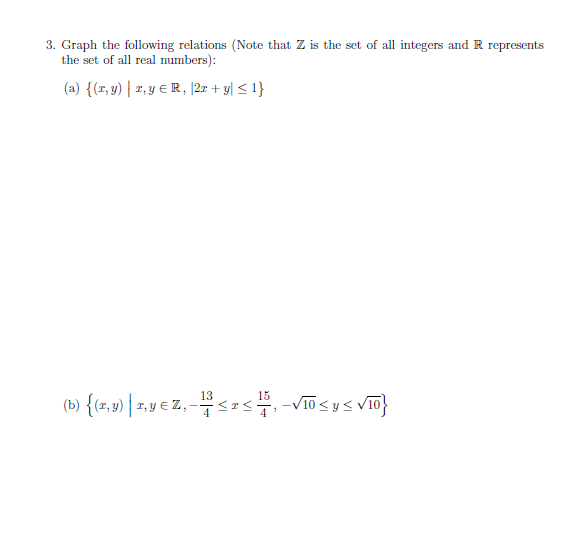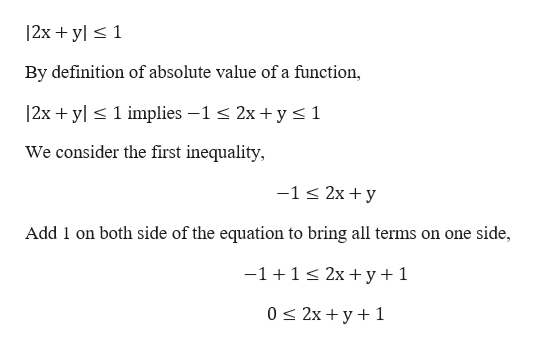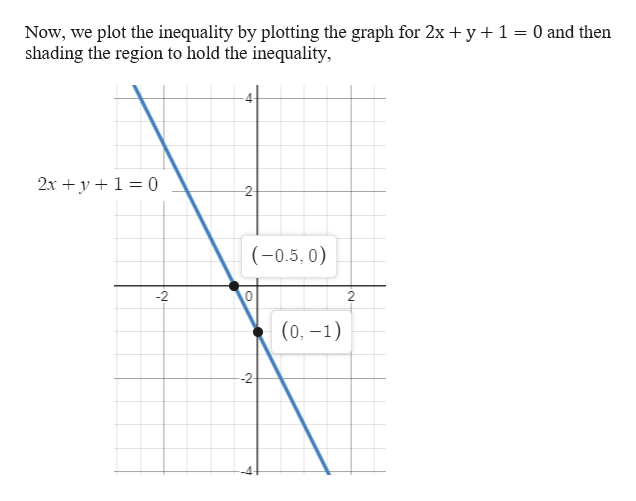# 3. Graph the following relations (Note that Z is the set of all integers and R representsthe set of all real numbers):(a) {(r,y),yR, |2 + y] < 1}1315(b) {(x, y)|,y Z,-V10 y104

Question

practice question 3help_outlineImage Transcriptionclose3. Graph the following relations (Note that Z is the set of all integers and R represents the set of all real numbers): (a) {(r,y),yR, |2 + y] < 1} 13 15 (b) {(x, y)|,y Z, -V10 y10 4 fullscreen
check_circle

Step 1

Hey, since there are multiple subparts posted, we will answer first question. If you want any specific question to be answered, then please submit that question only or specify the question number in your message

Given a relation

Step 2help_outlineImage Transcriptionclose2xy1 By definition of absolute value of a function, 12x yl1 implies -1 2xy1 We consider the first inequality, -1 2xy Add 1 on both side of the equation to bring all terms on one side, 2x+ y+ 1 -1+1 0 2xy 1 fullscreen
Step 3

To plot the g...help_outlineImage TranscriptioncloseNow, we plot the inequality by plotting the graph for 2x y 1 shading the region to hold the inequality, 0 and then 2x y 0 2- (-0.5, 0) 2 2 (0,-1) -2 fullscreen

### Want to see the full answer?

See Solution

#### Want to see this answer and more?

Solutions are written by subject experts who are available 24/7. Questions are typically answered within 1 hour.*

See Solution
*Response times may vary by subject and question.
Tagged in

### Equations and In-equations